1.A ray of red light enters a semi-circular glass block normal to the curved surface.Which diagram correctly shows the partial reflection and refraction of the ray?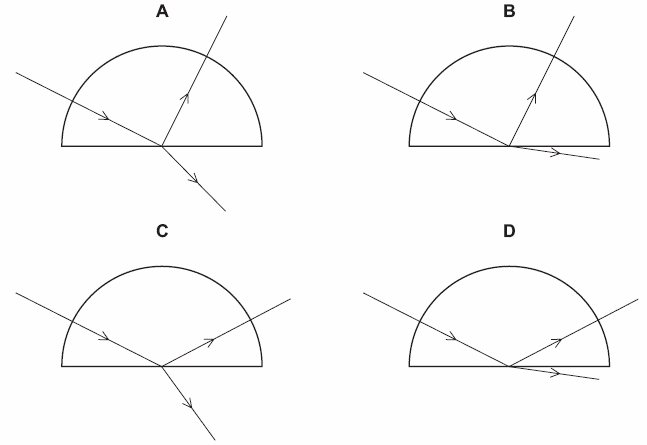2. A ripple tank is used to demonstrate refraction of plane water waves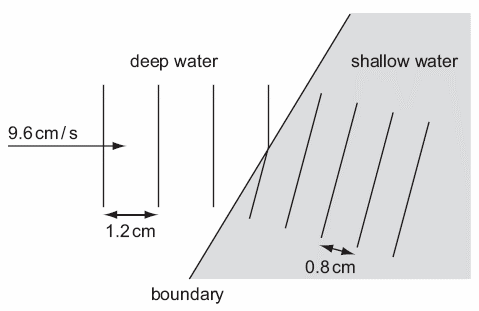Waves in deep water have a wavelength of 1.2 cm and a speed of 9.6 cm / s. The wavelength of the waves in shallow water is 0.8 cm. What is the speed of the waves in the shallow water?

3. The diagram shows a ray of light travelling from X. Angle P is less than the critical angle. In which direction does the ray continue?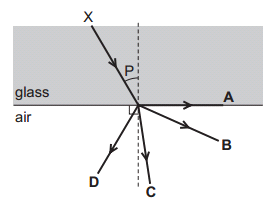4. The diagram shows the refraction of water waves in a ripple tank. The water is shallower above the glass sheet.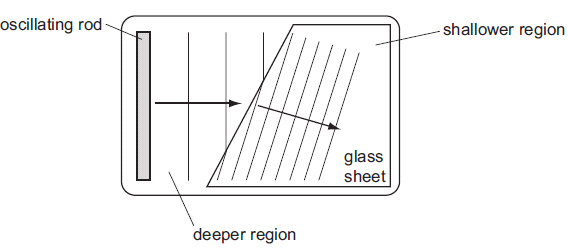When crossing into the shallower region, what is the effect on the frequency and on the speed of the waves?

5. A ray of light passes into a glass block of refractive index 1.5.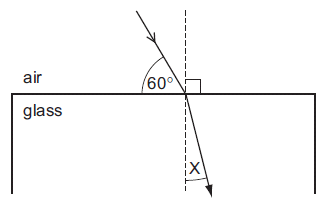What is the value of the angle marked X?

A.   19.50

B.   25.00

C.   35.30

D.   48.60

6. When a wave crosses the boundary between two media, which one of the following properties of the wave does not change?

7. On which one of the following graphs is the wavelength ë and the amplitude a of a wave correctly represented?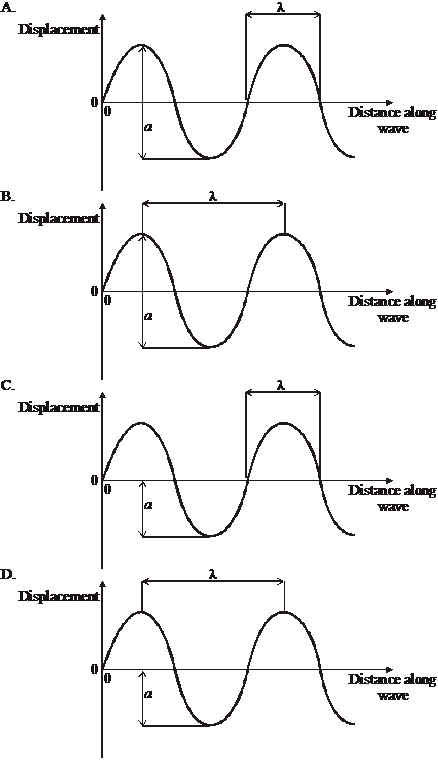8. The variation with time t of the separate displacements d of a point in a medium due to two waves is shown below.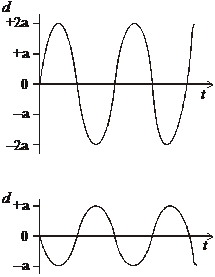The waves are superposed. Which of the following diagrams shows the variation with time t of the resultant displacement d of the point in the medium?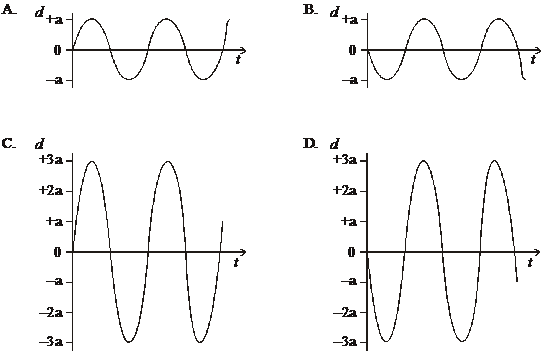9. . Sound waves move faster in warm air than in cold air. The diagram below shows plane waves in cold air moving towards a boundary with warm air.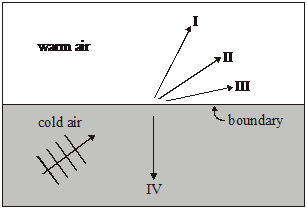Which of the arrows shows the possible direction of waves after reaching the boundary?

10. . Which diagram best shows diffraction of plane wavefronts at a single slit?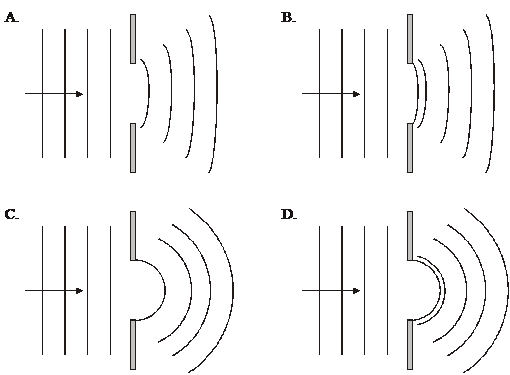Question 1 of 10